# RS Aggarwal Solutions for Class 7 Maths Exercise 6A Chapter 6 Algebraic Expressions

RS Aggarwal Solutions for Class 7 Maths Exercise 6A Chapter 6 Algebraic Expressions in simple PDF are available here. This exercise of RS Aggarwal Solutions for Class 7 Chapter 6 has the topics related to constants and variables. A symbol having a fixed numerical value is constant, whereas a symbol which takes various numerical values is variables. The exercise also contains addition and subtraction of algebraic expressions. Students are suggested to try solving the questions from RS Aggarwal Solutions for Class 7 Maths Chapter 6 Algebraic Expressions.

## Download the PDF of RS Aggarwal Solutions For Class 7 Maths Chapter 6 Algebraic Expressions – Exercise 6A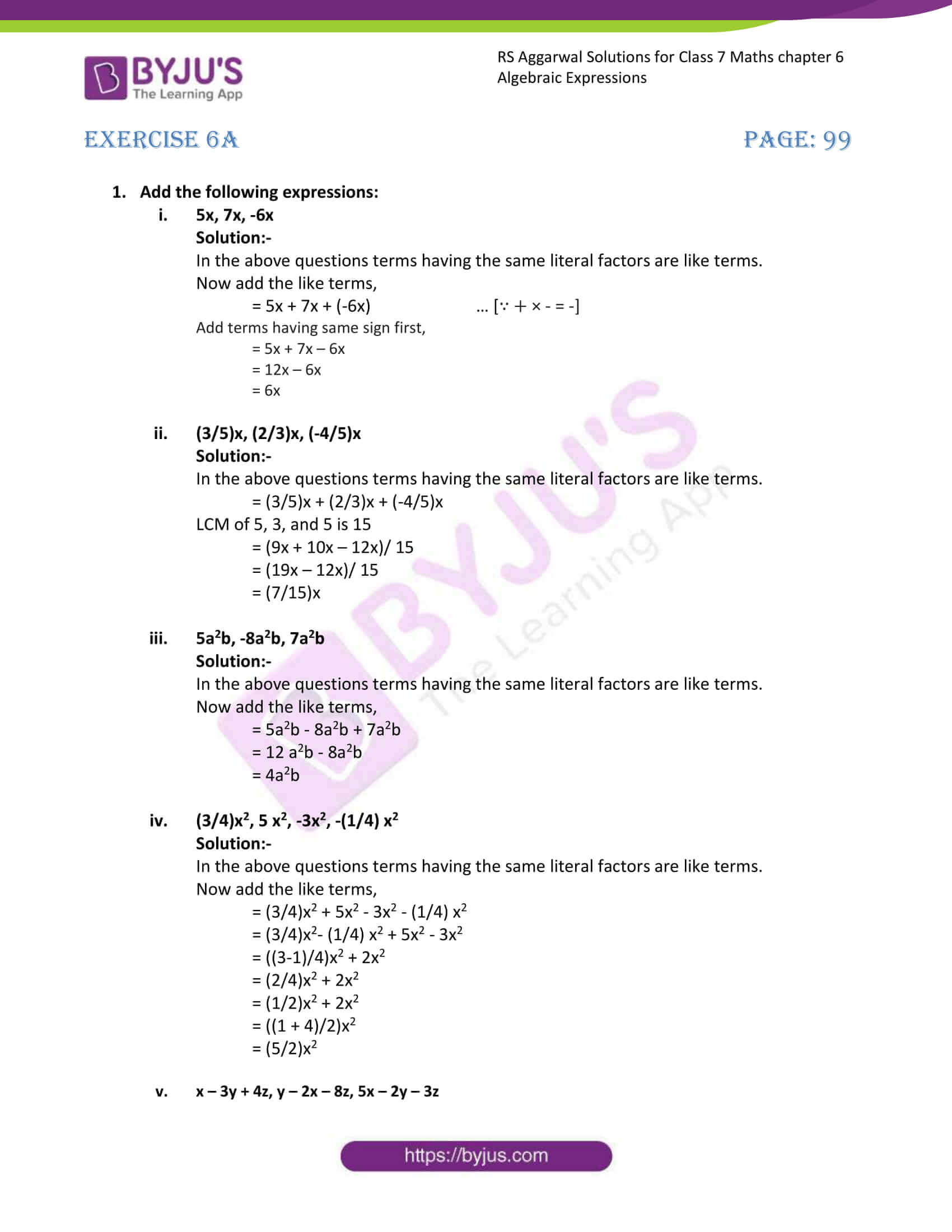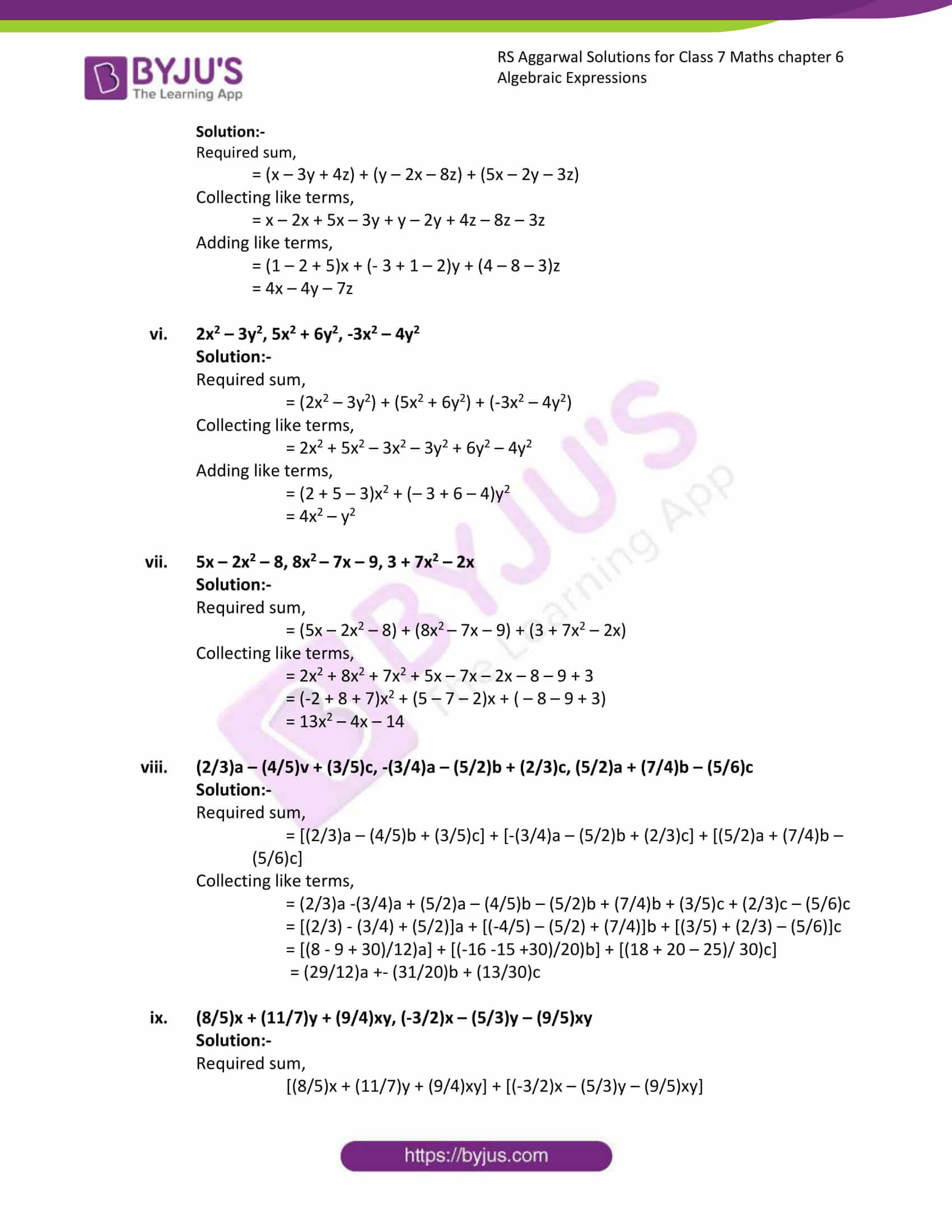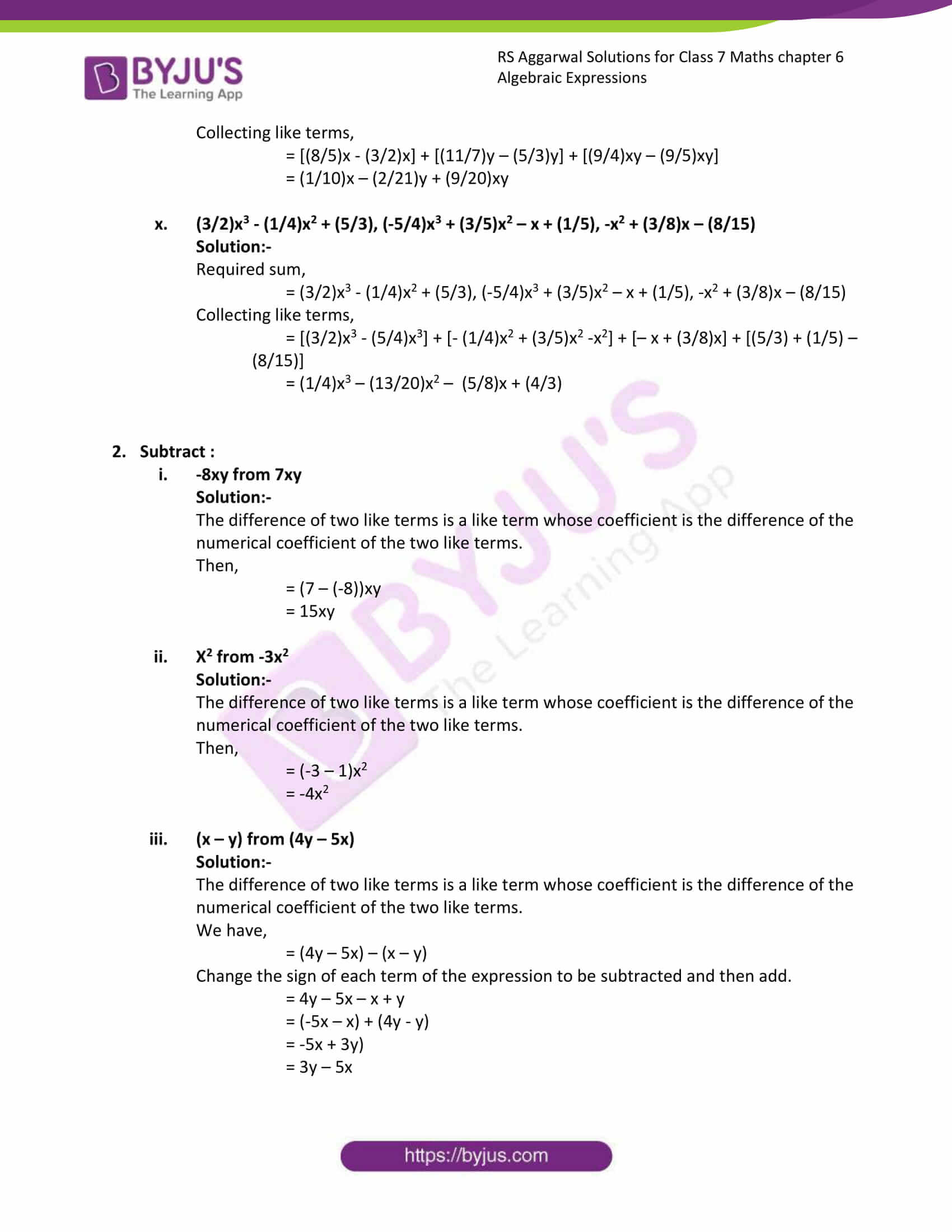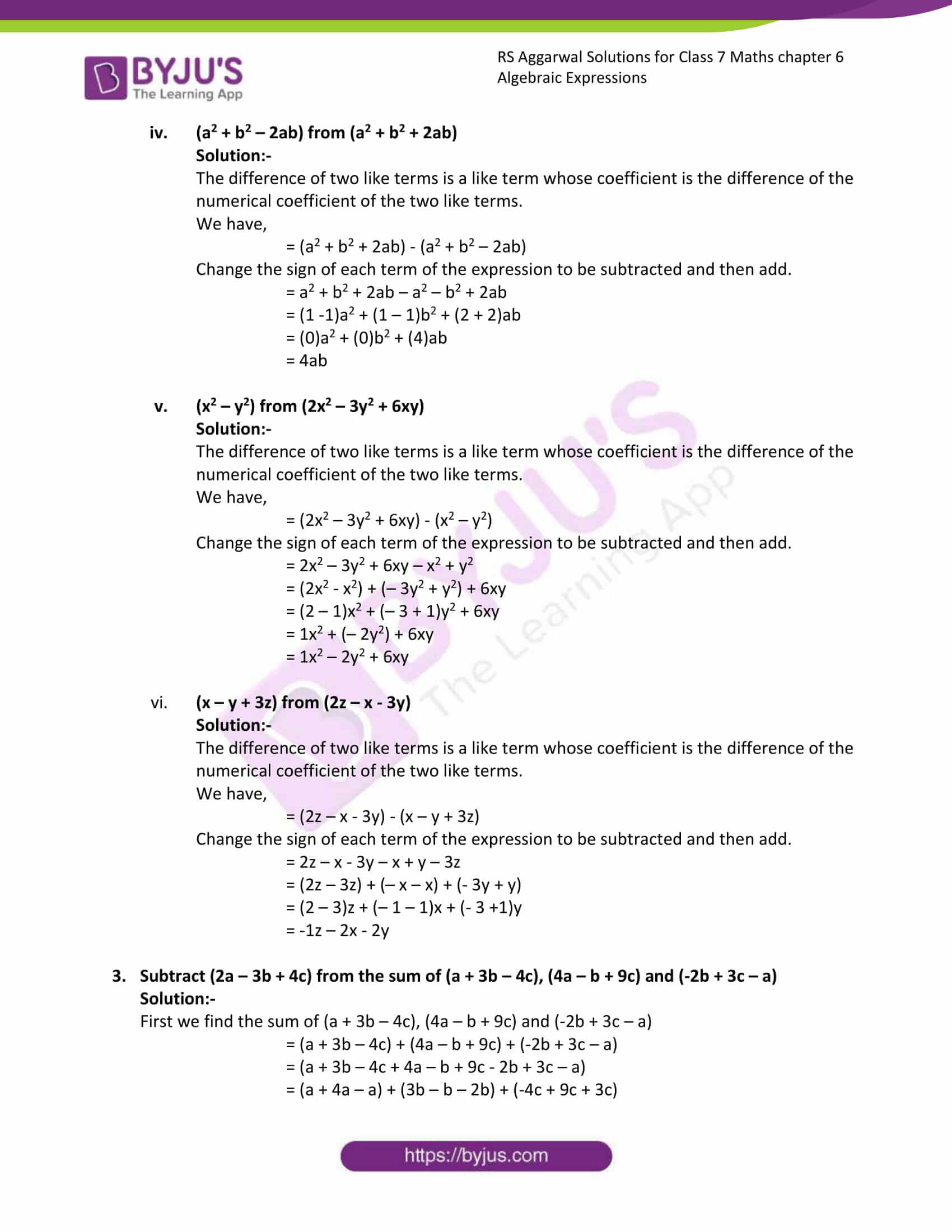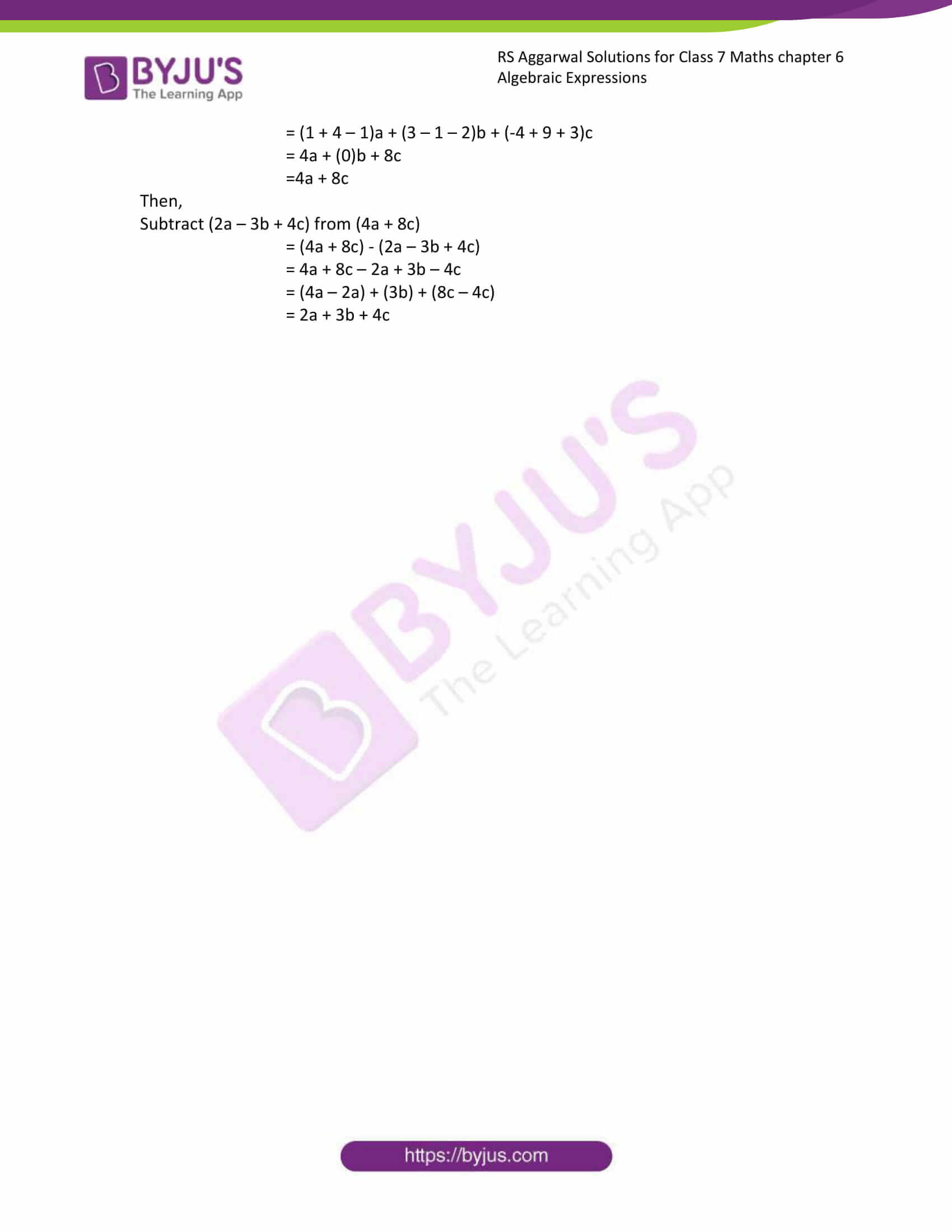### Access answers to Maths RS Aggarwal Solutions for Class 7 Chapter 6 – Algebraic Expressions Exercise 6A

(i) 5x, 7x, -6x

Solution:-

In the above questions terms having the same literal factors are like terms.

= 5x + 7x + (-6x) … [∵ + × – = -]

Add terms having same sign first,

= 5x + 7x – 6x

= 12x – 6x

= 6x

(ii) (3/5)x, (2/3)x, (-4/5)x

Solution:-

In the above questions terms having the same literal factors are like terms.

= (3/5)x + (2/3)x + (-4/5)x

LCM of 5, 3, and 5 is 15

= (9x + 10x – 12x)/ 15

= (19x – 12x)/ 15

= (7/15)x

(iii) 5a2b, -8a2b, 7a2b

Solution:-

In the above questions terms having the same literal factors are like terms.

= 5a2b – 8a2b + 7a2b

= 12 a2b – 8a2b

= 4a2b

(iv) (3/4)x2, 5 x2, -3x2, -(1/4) x2

Solution:-

In the above questions terms having the same literal factors are like terms.

= (3/4)x2 + 5x2 – 3x2 – (1/4) x2

= (3/4)x2– (1/4) x2 + 5x2 – 3x2

= ((3-1)/4)x2 + 2x2

= (2/4)x2 + 2x2

= (1/2)x2 + 2x2

= ((1 + 4)/2)x2

= (5/2)x2

(v) x – 3y + 4z, y – 2x – 8z, 5x – 2y – 3z

Solution:-

Required sum,

= (x – 3y + 4z) + (y – 2x – 8z) + (5x – 2y – 3z)

Collecting like terms,

= x – 2x + 5x – 3y + y – 2y + 4z – 8z – 3z

= (1 – 2 + 5)x + (- 3 + 1 – 2)y + (4 – 8 – 3)z

= 4x – 4y – 7z

(vi) 2x2 – 3y2, 5x2 + 6y2, -3x2 – 4y2

Solution:-

Required sum,

= (2x2 – 3y2) + (5x2 + 6y2) + (-3x2 – 4y2)

Collecting like terms,

= 2x2 + 5x2 – 3x2 – 3y2 + 6y2 – 4y2

= (2 + 5 – 3)x2 + (– 3 + 6 – 4)y2

= 4x2 – y2

(vii) 5x – 2x2 – 8, 8x2 – 7x – 9, 3 + 7x2 – 2x

Solution:-

Required sum,

= (5x – 2x2 – 8) + (8x2 – 7x – 9) + (3 + 7x2 – 2x)

Collecting like terms,

= 2x2 + 8x2 + 7x2 + 5x – 7x – 2x – 8 – 9 + 3

= (-2 + 8 + 7)x2 + (5 – 7 – 2)x + ( – 8 – 9 + 3)

= 13x2 – 4x – 14

(viii) (2/3)a – (4/5)v + (3/5)c, -(3/4)a – (5/2)b + (2/3)c, (5/2)a + (7/4)b – (5/6)c

Solution:-

Required sum,

= [(2/3)a – (4/5)b + (3/5)c] + [-(3/4)a – (5/2)b + (2/3)c] + [(5/2)a + (7/4)b – (5/6)c]

Collecting like terms,

= (2/3)a -(3/4)a + (5/2)a – (4/5)b – (5/2)b + (7/4)b + (3/5)c + (2/3)c – (5/6)c

= [(2/3) – (3/4) + (5/2)]a + [(-4/5) – (5/2) + (7/4)]b + [(3/5) + (2/3) – (5/6)]c

= [(8 – 9 + 30)/12)a] + [(-16 -15 +30)/20)b] + [(18 + 20 – 25)/ 30)c]

= (29/12)a +- (31/20)b + (13/30)c

(ix) (8/5)x + (11/7)y + (9/4)xy, (-3/2)x – (5/3)y – (9/5)xy

Solution:-

Required sum,

[(8/5)x + (11/7)y + (9/4)xy] + [(-3/2)x – (5/3)y – (9/5)xy]

Collecting like terms,

= [(8/5)x – (3/2)x] + [(11/7)y – (5/3)y] + [(9/4)xy – (9/5)xy]

= (1/10)x – (2/21)y + (9/20)xy

(x) (3/2)x3 – (1/4)x2 + (5/3), (-5/4)x3 + (3/5)x2 – x + (1/5), -x2 + (3/8)x – (8/15)

Solution:-

Required sum,

= (3/2)x3 – (1/4)x2 + (5/3), (-5/4)x3 + (3/5)x2 – x + (1/5), -x2 + (3/8)x – (8/15)

Collecting like terms,

= [(3/2)x3 – (5/4)x3] + [- (1/4)x2 + (3/5)x2 -x2] + [– x + (3/8)x] + [(5/3) + (1/5) – (8/15)]

= (1/4)x3 – (13/20)x2 – (5/8)x + (4/3)

2. Subtract :

(i) -8xy from 7xy

Solution:-

The difference of two like terms is a like term whose coefficient is the difference of the numerical coefficient of the two like terms.

Then,

= (7 – (-8))xy

= 15xy

(ii) X2 from -3x2

Solution:-

The difference of two like terms is a like term whose coefficient is the difference of the numerical coefficient of the two like terms.

Then,

= (-3 – 1)x2

= -4x2

(iii) (x – y) from (4y – 5x)

Solution:-

The difference of two like terms is a like term whose coefficient is the difference of the numerical coefficient of the two like terms.

We have,

= (4y – 5x) – (x – y)

Change the sign of each term of the expression to be subtracted and then add.

= 4y – 5x – x + y

= (-5x – x) + (4y – y)

= -5x + 3y)

= 3y – 5x

(iv) (a2 + b2 – 2ab) from (a2 + b2 + 2ab)

Solution:-

The difference of two like terms is a like term whose coefficient is the difference of the numerical coefficient of the two like terms.

We have,

= (a2 + b2 + 2ab) – (a2 + b2 – 2ab)

Change the sign of each term of the expression to be subtracted and then add.

= a2 + b2 + 2ab – a2 – b2 + 2ab

= (1 -1)a2 + (1 – 1)b2 + (2 + 2)ab

= (0)a2 + (0)b2 + (4)ab

= 4ab

(v) (x2 – y2) from (2x2 – 3y2 + 6xy)

Solution:-

The difference of two like terms is a like term whose coefficient is the difference of the numerical coefficient of the two like terms.

We have,

= (2x2 – 3y2 + 6xy) – (x2 – y2)

Change the sign of each term of the expression to be subtracted and then add.

= 2x2 – 3y2 + 6xy – x2 + y2

= (2x2 – x2) + (– 3y2 + y2) + 6xy

= (2 – 1)x2 + (– 3 + 1)y2 + 6xy

= 1x2 + (– 2y2) + 6xy

= 1x2 – 2y2 + 6xy

(vi) (x – y + 3z) from (2z – x – 3y)

Solution:-

The difference of two like terms is a like term whose coefficient is the difference of the numerical coefficient of the two like terms.

We have,

= (2z – x – 3y) – (x – y + 3z)

Change the sign of each term of the expression to be subtracted and then add.

= 2z – x – 3y – x + y – 3z

= (2z – 3z) + (– x – x) + (- 3y + y)

= (2 – 3)z + (– 1 – 1)x + (- 3 +1)y

= -1z – 2x – 2y

3. Subtract (2a – 3b + 4c) from the sum of (a + 3b – 4c), (4a – b + 9c) and (-2b + 3c – a)

Solution:-

First we find the sum of (a + 3b – 4c), (4a – b + 9c) and (-2b + 3c – a)

= (a + 3b – 4c) + (4a – b + 9c) + (-2b + 3c – a)

= (a + 3b – 4c + 4a – b + 9c – 2b + 3c – a)

= (a + 4a – a) + (3b – b – 2b) + (-4c + 9c + 3c)

= (1 + 4 – 1)a + (3 – 1 – 2)b + (-4 + 9 + 3)c

= 4a + (0)b + 8c

=4a + 8c

Then,

Subtract (2a – 3b + 4c) from (4a + 8c)

= (4a + 8c) – (2a – 3b + 4c)

= 4a + 8c – 2a + 3b – 4c

= (4a – 2a) + (3b) + (8c – 4c)

= 2a + 3b + 4c

### Access other exercises of RS Aggarwal Solutions For Class 7 Chapter 6 – Algebraic Expressions

Exercise 6D Solutions